Open in App
Not now

# Potential Energy

• Last Updated : 26 Apr, 2021

Potential energy is the energy gained by an object, just because of its position relative to other objects, stresses within itself, its electric charge, or other factors. Why a stone falling from a building crashes everything that comes in its way. Why an arrow moves faster when released with more tension in the rubber. It seems that in these examples, stone or arrow has some energy. From where this energy comes?

Let’s understand with an example.

Take a rubber band and pull it on both ends. The band stretches. Now release the band at one of the ends. What happens?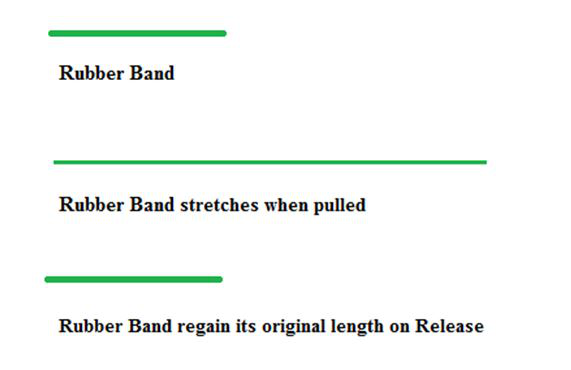The band will tend to regain its original length. Obviously, the band had acquired energy in its stretched position. How did it acquire energy when stretched?

In the above situations, the energy gets stored in the object due to the work done. The energy transferred to the object is stored as potential energy if it does not change the velocity or speed of the object. We transfer energy when we stretch a rubber band.

The energy transferred to the band equals to its potential energy. The potential energy possessed by the object equals to the energy present in it by virtue of its position or configuration.

An object can gain energy because of its position.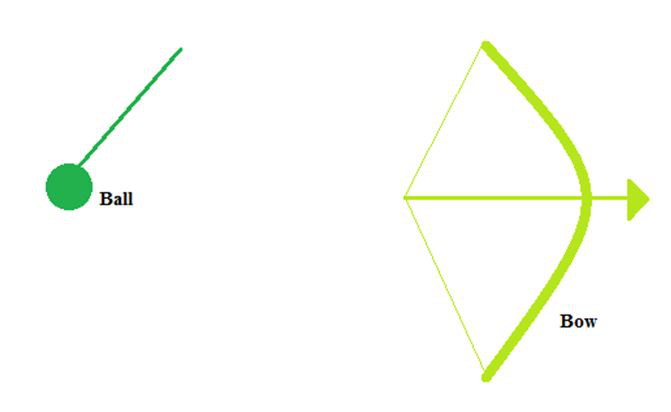The heavy ball of a machine is storing energy when it is held at a high elevated position. This stored energy of the position is referred to as potential energy.

In Second example, a drawn bow is able to store energy as the result of its position. When assuming its initial position (i.e., when not drawn), there is zero energy stored in the bow. When its position is changed from its initial equilibrium position, the bow is able to store energy caused by its position. This stored energy due to the position of the object is referred to as potential energy. Potential energy is the stored energy of an object caused by the position possessed.

In this chapter, we will read about potential energy, gravitational potential energy, formulae of potential energy and will solve some examples to understand better.

The energy of an object increases when it is raised through a height. This energy is stored because work is done on the object against gravity while it is being raised. The energy stored in such an object equals the gravitational potential energy.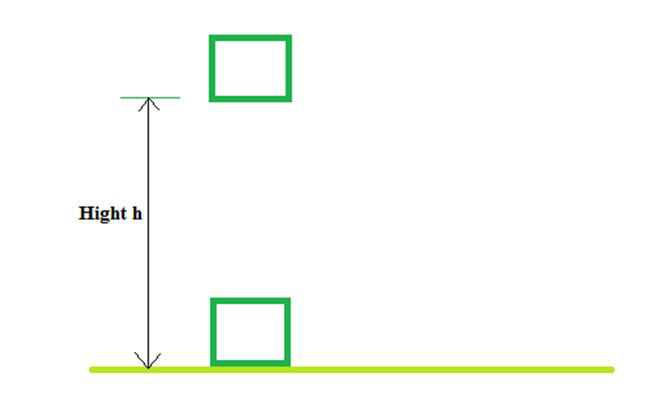When work is done on it, energy can get stored in an entity. For example, stretching a rubber string. The energy that is possessed by a body under its configuration or change in position is known as Potential Energy.

Consider an object of mass, m. It is being raised through a height, h from the ground. To do this a force is required. The minimum force required to raise the object is always equal to the weight of the object i.e. F = mg.

The object stores energy equal to the work done on it. Suppose the work done on the object against gravity be W.

Work done, W = force × displacement

W = mg × h

W = mgh

Since work done on the object is equal to mgh, an energy equal to mgh units is stored in the object. This is the potential energy (EP) of the object.

Ep = mgh

### Types of Potential Energy

There are two main types of potential energy, and they are:

• Gravitational Potential Energy
• Elastic Potential Energy

### Gravitational Potential Energy

The gravitational potential energy of an object is defined as the energy possessed by an object that rose to a certain height against gravity. We can express gravitational energy with the help of the following example:

Let’s take an object of mass m at a height h from the ground, as shown in the figure. Now, as we know, the force required to raise the object is equal to m × g of the object.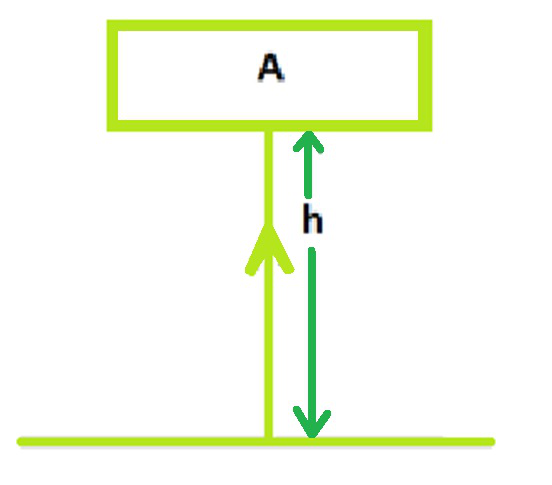As per the figure, an object raised against the force of gravity. Some amount of work W is done on it.

Work done = force × displacement.

So,

W = m × g × h

W = mgh

According to the law of conservation of energy, work done on the object is equal to mgh, the energy gained by the object = mgh, which in this case is the potential energy E.

E of an object raised to a height h above the ground = m×g×h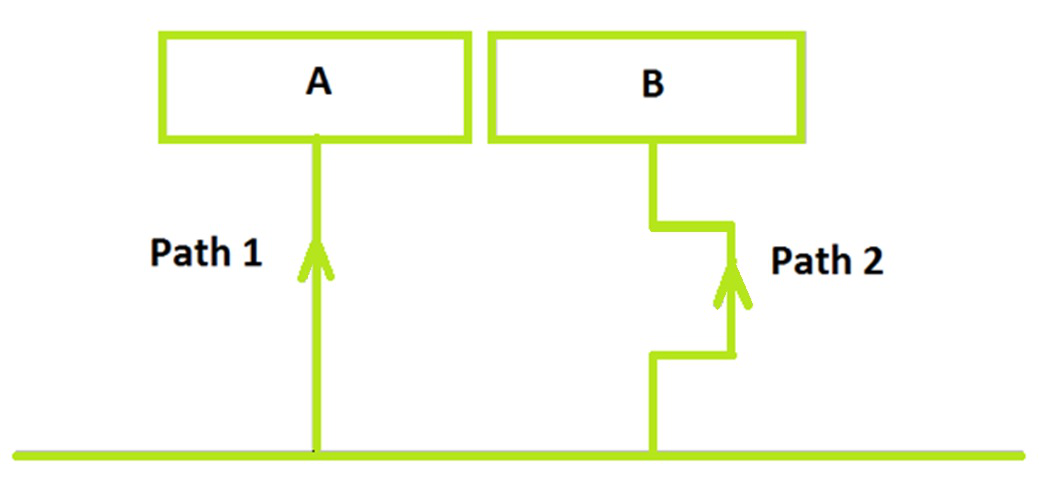### Elastic Potential Energy

Elastic potential energy is the energy that is stored in objects. It can be compressed or stretched such as rubber bands, trampoline, and bungee cords. The object that can stretch more, has more elastic potential energy.

Examples:

• A twisted rubber band that powers a toy plane
• An archer’s stretched bow
• A bent driver’s board just before a diver dives in
• Coil spring of a wind-up clock

Elastic potential energy can be calculated using the following formula:

U = (1/2)kx2

Where,

U is the elastic potential energy

k is the spring force constant

x is the string stretch length in m

### Sample Problems

Problem 1. Find the potential energy of an object of mass 10 kg when it is raised at a height of 6 m above the ground. Take, g = 10 m s–2.

Solution:

Given

Mass of the object m = 10 Kg

Height above the ground h = 6 m

Find Out

Potential energy Ep =?

We Know that

Ep = mgh

Ep = 10 × 10 × 6

Ep = 600 J

Problem 2. A Ball of mass 22 kg is at a certain height above the ground. If the potential energy of the object is 880 J, find the height at which the object is with respect to the ground. Take, g = 10 m s–2.

Solution:

Given,

Mass of the Ball, m = 22 kg,

Potential energy, Ep = 880 J.

Find Out

Height above the ground h = ?

We Know that

Ep = mgh

880 = 22 × 10 × h

h = 880/220

h = 4 m.

The height of the object is 4 m.

Problem 3. An asteroid is coming towards the earth. Its height above the ground is 1000 km. Its estimated potential energy is almost 4× 1015 J. Find out the mass of asteroid.

Solution:

Given

Potential energy Ep = 3.72 × 1013 J,

Height of the asteroid, h = 1000Km = 106 m

Find Out:

Mass of the asteroid, m =?

We know that

Ep = mgh

3.72 × 1013= m × 10 × 106

m= 3.72 × 106 Kg

Mass of asteroid is 3.72 × 106 Kg

Problem 4. A spring has a strength length of 0.7 m. Take k= 16. What is its elastic potential energy?

Solution:

Given

k = 7

Length in m = .4 m

Find Out

Elastic potential energy =?

U = ½ kx2

U = ½ × 16× 0.7× 0.7

U = 3.92 J

Problem 5. A spring with spring force constant k= 12 has elastic potential energy of 24 J. Find out its length in m.

Solution:

Given,

k = 12

U = 24 J

Length in m =?

U = ½ kx2

24 = ½ ×12 × x2

x = 2 m

My Personal Notes arrow_drop_up